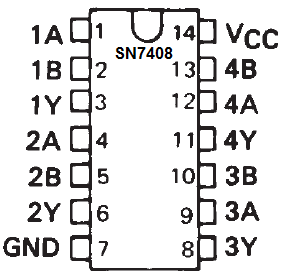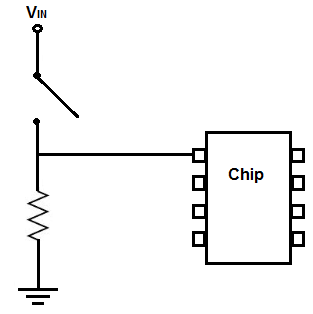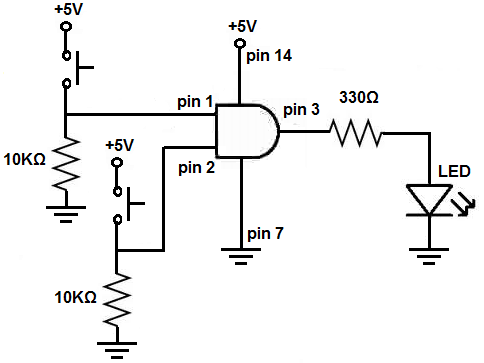﻿ How to Connect a Pull Down Resistor ﻿# How to Connect a Pull Down ResistorIn this project, we will show how to wire up a pull down resistor to a circuit.

A pull down resistor is a resistor that makes the pin of a chip normally LOW and then turns it HIGH when switched on.

Pull up or pull down resistors are normally connected to the pins of chips so that they can define the state of the pins.

Pins of chips can be in any of 3 states.

They can be LOW, meaning a voltage near 0V (or below half of the voltage fed into the power supply of the chip) is fed into the pin.

Pins can be HIGH, meaning a voltage above half of the voltage fed into the chip's power supply is fed into the pin.

Or pins can be floating, meaning they are unconnected to either a LOW or HIGH voltage. In this case, the pin may go randomly between LOW or HIGH. Pins may float because they have no clear defined voltage signal going into it. Things such as external noise may allow it to haphazardly take on both values.

It is undesired for pins to float. And this is why we normally want the signal going into a pin to be clearly defined. That way, we know for sure whether it is LOW or HIGH. And this is why we use pull up and pull down resistors.

Pull up resistors pull up a pin to a HIGH state.

Pull down resistors pull down a pin to a LOW state.

When connected to a switch such a pushbutton, the states of the pins can then be switched to the other when switched on.

We will show now how to connect a pull down resistor to a pin of a chip so that a pin can be normally LOW and then switch high when a switch such as a pushbutton is pushed down.

One of the best circuits to test pull down resistors on are logic chip circuits. Logic chips are chips that work purely based on LOW or HIGH signals.

An AND gate is a logic chip that outputs a HIGH signal only when both inputs of the gate are HIGH. If either or both inputs are LOW, the AND gate will output a LOW signal and the load on the output will not turn on.

We will connect both inputs going into an AND gate to pull down resistors. This will make the pins normally LOW. So normally the inputs will be at a logic LOW level. So the output will be LOW and the load will not be powered on. When the pushbuttons connected to the resistors are pushed down, then the pin will be drawn to a HIGH state. When both pushbuttons are pushed down and are, thus, HIGH, then the AND gate will have a HIGH output and turn on the load on its output pin.

This circuit will perfectly demonstrate pull down resistors.

Components Needed

• SN7408 AND Gate Chip
• 2 10KΩ Resistor
• 2 Pushbuttons
• LED
• 330Ω Resistor

The SN7408 quad AND gate chip can be obtained very cheaply from a number of online retailers for just a few cents. One place it can be obtained from is Tayda Electronics at the following link: Tayda Electronics- 7408 Quad 2-Input AND Gate IC. However, it is a very popular chip and many electronics parts suppliers have them.

The pinout of the 7408 is shown below, so that you can see how to connect it in the circuit.Each AND gate has 2 input pins and 1 output pin.

We will use 2 pushbuttons as our inputs to the AND gate.

The value we will use for the pull down resistors are 10KΩ.

The other components we need are the LED and the 330Ω resistor in series to limit current to the LED so that it doesn't burn out.

### Pull Down Resistor Circuit Theory

The perfect model of a pull-down resistor circuit is shown below.This pull down resistor circuit can be used exactly as is for every pull down resistor circuit.

The pin of this chip will be LOW normally and when the switch is closed becomes HIGH.

How this circuit works is the pin of the chip, when the switch is open, is connected directly to ground. Therefore, it is at a logic LOW level. When the switch is closed, the voltage from the power supply falls across the resistor. Thus, the pin of the chip is drawn to a HIGH state.

We will use this model with our circuit below.

### AND Gate Circuit Using Pull down Resistors

The schematic diagram of the AND gate circuit using 2 pull down resistors at the inputs to the gate is shown below.Below is the breadboard schematic version of the above circuit so that you can see the exact wiring of the circuit to the 4011 chip.First and foremost, we must give power to the chip. We will feed it 5V of power, so we give +5V to pin 14 and we connect pin 7 to GND. This establishes power to the chip.

Next we connect pull down resistors to each of the inputs of the AND gate. A pull-down resistor circuit first has a pushbutton connected to positive voltage and then a 10KΩ resistor connected to ground. When the pushbutton is not pushed, each of the pins of the AND gate will be LOW. When a pushbutton is pressed down, the resistor gets the voltage dropped across, so the pin of the AND gate will now be HIGH. For AND gate logic, both inputs must be HIGH for an output of HIGH. Therefore, you must press down on both pushbuttons in order for the circuit to work.

And this illustrates the greatness and extreme usefulness of pull down resistors. When working with chips, they allow a chip to have a pin that is normally LOW, which then can be switched HIGH. Otherwise, there would be no way of doing it. So through this circuit, the inputs will be normally LOW, so that the output is off initially. But when both pushbuttons are pressed, the inputs are HIGH and now the output will be HIGH.

Related Resources

How to Connect a Pull Up Resistor